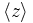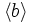# Binary octahedral group

View a complete list of particular groups (this is a very huge list!)[SHOW MORE]

## Definition

The binary octahedral group can be defined in the following equivalent ways:

1. It is the Schur cover of symmetric group:S4 of type "-" which in symbols means it is the group$2 \cdot S_4^-$.
2. It is a binary von Dyck group with parameters$(2,3,4)$, i.e., it has the presentation:$\! \langle a,b,c \mid a^4 = b^3 = c^2 = abc\rangle$
We denote the element$a^4 = b^3 = c^2$ as$z$. This element has order two.

## Arithmetic functions

Function Value Similar groups Explanation for function value
order (number of elements, equivalently, cardinality or size of underlying set) 48 groups with same order As$2 \cdot S_n^-, n = 4$:$2(n!) = 2(4!) = 2(24) = 48$
As binary von Dyck group with parameters$(p,q,r) = (2,3,4)$:$\frac{4}{1/p + 1/q + 1/r - 1} = \frac{4}{1/2 + 1/3 + 1/4 - 1} = \frac{4}{1/12} = 48$
exponent of a group 24 groups with same order and exponent of a group | groups with same exponent of a group As binary von Dyck group with parameters$(p,q,r) = (2,3,4)$:$2 \operatorname{lcm} \{ p,q,r \} = 2 \operatorname{lcm} \{ 2,3,4 \} = 2(12) = 24$
derived length 4 groups with same order and derived length | groups with same derived length derived subgroup is isomorphic to SL(2,3). Derived subgroup of that is isomorphic to quaternion group. Derived subgroup of that is isomorphic to cyclic group:Z2, which is abelian.
nilpotency class -- not a nilpotent group.
Frattini length 2 groups with same order and Frattini length | groups with same Frattini length Intersection of Frattini subgroups is center of binary octahedral group which is cyclic of order two.
minimum size of generating set 2 groups with same order and minimum size of generating set | groups with same minimum size of generating set
subgroup rank of a group 2 groups with same order and subgroup rank of a group | groups with same subgroup rank of a group --
max-length of a group 5 groups with same order and max-length of a group | groups with same max-length of a group

### Arithmetic functions of a counting nature

Function Value Similar groups Explanation for function value
number of conjugacy classes 8 groups with same order and number of conjugacy classes | groups with same number of conjugacy classes As binary von Dyck group with parameters$(p,q,r) = (2,3,4)$:$p + q + r - 1 = 2 + 3 + 4 - 1 = 8$
See element structure of binary octahedral group
Note also that the number of conjugacy classes equals that of the other double cover of$S_4$, namely general linear group:GL(2,3)
number of subgroups 35 groups with same order and number of subgroups | groups with same number of subgroups See subgroup structure of binary octahedral group
number of conjugacy classes of subgroups 13 groups with same order and number of conjugacy classes of subgroups | groups with same number of conjugacy classes of subgroups See subgroup structure of binary octahedral group

## Group properties

Property Satisfied Explanation
Abelian group No
Nilpotent group No
Metacyclic group No
Supersolvable group No
Solvable group Yes Length four.
T-group No
HN-group No
Monolithic group Yes The center of order two is the unique minimal normal subgroup.

## Subgroups

Further information: subgroup structure of binary octahedral group

### Quick summary

Item Value
Number of subgroups 35
Number of conjugacy classes of subgroups 13
Number of automorphism classes of subgroups 13
Isomorphism classes of Sylow subgroups and the corresponding Sylow numbers and fusion systems 2-Sylow: generalized quaternion group:Q16, Sylow number 3, fusion system ?
3-Sylow: cyclic group:Z3, Sylow number 4
maximal subgroups Maximal subgroups are: derived subgroup of binary octahedral group (isomorphic to SL(2,3), order 24), 2-Sylow subgroups of binary octahedral group (isomorphic to Q16, order 16), and subgroups of order 12 isomorphic to dicyclic group:Dic12

### Table classifying subgroups up to automorphisms

TABLE SORTING AND INTERPRETATION: Note that the subgroups in the table below are sorted based on the powers of the prime divisors of the order, first covering the smallest prime in ascending order of powers, then powers of the next prime, then products of powers of the first two primes, then the third prime, then products of powers of the first and third, second and third, and all three primes. The rationale is to cluster together subgroups with similar prime powers in their order. The subgroups are not sorted by the magnitude of the order. To sort that way, click the sorting button for the order column. Similarly you can sort by index or by number of subgroups of the automorphism class.
Automorphism class of subgroups Representative subgroup (full list if small, generating set if large) Isomorphism class Order of subgroups Index of subgroups Number of conjugacy classes (=1 iff automorph-conjugate subgroup) Size of each conjugacy class (=1 iff normal subgroup) Total number of subgroups (=1 iff characteristic subgroup) Quotient group (if it exists)
trivial subgroup$\{ e \}$ trivial group 1 48 1 1 1 binary octahedral group
center of binary octahedral group$\langle z \rangle$ cyclic group:Z2 2 24 1 1 1 symmetric group:S4
3-Sylow subgroups of binary octahedral group$\langle b^2 \rangle$ cyclic group:Z3 3 16 1 4 4 --
cyclic subgroups of order four$\langle c \rangle$ cyclic group:Z4 4 12 1 --
cyclic subgroups of order four$\langle a^2 \rangle$ cyclic group:Z4 4 12 1 --
cyclic subgroups of order six$\langle b \rangle$ cyclic group:Z6 6 8 1 4 4 --
quaternion subgroup of one type$\langle aca^{-3},c \rangle$ quaternion group 8 6 --

(remaining items to be transcribed into table later)

1. The quaternion group of the form PLACEHOLDER FOR INFORMATION TO BE FILLED IN: [SHOW MORE]. Isomorphic to quaternion group.
2. The cyclic groups conjugate to$\langle a \rangle$. Isomorphic to cyclic group:Z8. (3)
3. The dicyclic group of order$12$, i.e., the binary von Dyck group$\Gamma(3,2,2)$. Isomorphic to dicyclic group:Dic12. (4)
4. The generalized quaternion group of order$16$. Isomorphic to generalized quaternion group. (3)
5. A unique subgroup of order$24$, isomorphic to special linear group:SL(2,3). (1)
6. The whole group. (1)

## GAP implementation

### Group ID

This finite group has order 48 and has ID 28 among the groups of order 48 in GAP's SmallGroup library. For context, there are 52 groups of order 48. It can thus be defined using GAP's SmallGroup function as:

SmallGroup(48,28)

For instance, we can use the following assignment in GAP to create the group and name it$G$:

gap> G := SmallGroup(48,28);

Conversely, to check whether a given group$G$ is in fact the group we want, we can use GAP's IdGroup function:

IdGroup(G) = [48,28]

or just do:

IdGroup(G)

to have GAP output the group ID, that we can then compare to what we want.